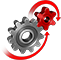# WolframSystemModeler

## Chuas's Circuit

Chua’s circuit is the simplest electronic circuit that displays chaotic behavior. Due to its simplicity, it is especially suitable as a demonstrative real-world example of a chaotic system.

### The ModelThe chaotic system of the Chua circuit consists of linear basic elements (capacitors, resistor, conductor, inductor) and a nonlinear resistor called Chua’s diode.

### Simulation & Animation2D phase portraits for the two capacitors in the Chua circuit.
The WSMLink linking SystemModeler to Mathematica provides a number of tools for model visualization. Here, some well‐chosen model equations from SystemModeler have been extracted in Mathematica and used to create a three‐dimensional parametric plot animation.
The animation is based on simulation results obtained from the model. As the plot evolves, it starts to show the characteristically chaotic "double scroll" attractor pattern.